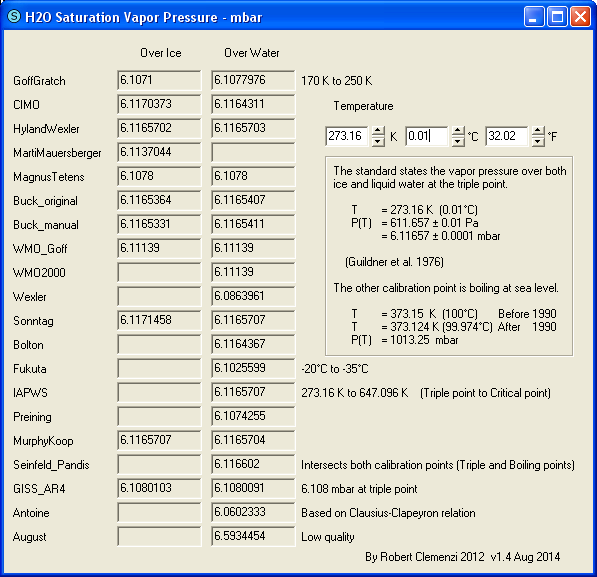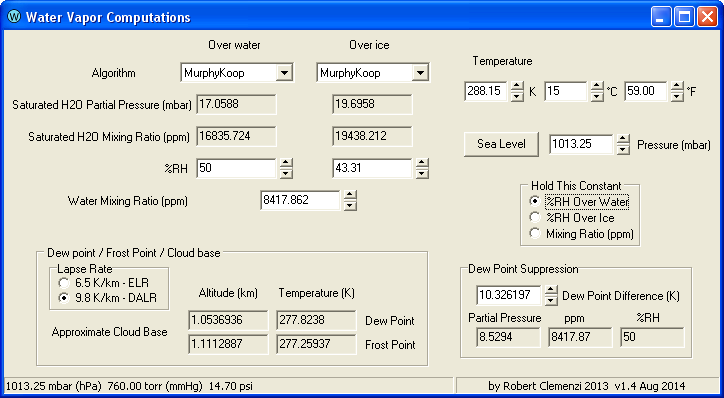### Water Vapor Programs

I have written several programs to help understand the problems computing relative humidity - zip. These programs implement 30 equations (algorithms) to compute saturated water vapor based only on the provided temperature.

Saturation_vapor_pressure.exe | Water_Vapor.exe | mcSaturationVaporPressure.pas | Numeric Fields | Versions

### Saturation_vapor_pressure.exe

There are many equations to compute the saturation water vapor pressure depending on the temperature. Saturation_vapor_pressure.exe shows the results of 30 algorithms for easy comparison - just set the temperature. (All 3 temperature fields are linked - just pick one.)From Review of the vapour pressures of ice and supercooled water for atmospheric applications by D. M. Murphy and T. Koop, 29 Dec 2006

 The vapour pressure of both ice and liquid water at the triple point is Pt = 611.657 ± 0.01 Pa at temperature Tt = 273.16 K (Guildner et al. 1976, Vapor pressure of water at its triple point. J. Res. Natl. Bur. Stand., 80A, 505–521)
This program shows that many algorithms don't intersect with this.

The second calibration point is a problem - ref

• Before 1990 (IPTS-68) , water at 1 atm (1013.25 mbar) boiled at 100°C (373.15 K) (by definition)
• After 1990 (ITS-90), the second calibration point for the temperature scale was changed to absolute zero (0.0 K) and the new boiling point of water at 1 atm was measured to be 99.974°C (373.124 K)

Perhaps 2 of the formulations have been modified to take this into account

• IAPWS - Their page is my reference for this change
• HylandWexler - This model was produced several years before the change occurred - 1983 vs 1990
Otherwise, as far as I can tell, none of the other saturation vapor pressure algorithms have been modified to take this into account. (It's been 24 years and counting.) Maybe it does not really matter since most of the algorithms don't intersect either boiling point.

By the way, there is no requirement that a good algorithm has to intersect one, or both, calibration points (though it would be nice). "Improved Magnus` form approximation of saturation vapor pressure" argues that a formulation that produces the smallest maximum error may be "better" than the algorithm with the smallest overall error (determined by, for example, a least squares fit) if the tail values are off by a large amount.

Both programs normally limit the temperature precision to only 2 decimal places. To use additional precision, type the value into the Kelvin field. The only time I have needed this is when checking the *new* boiling point of water at 1 atm - the old standard was 100°C and the new value is 99.974°C .. because the standard temperature scale was redefined in 1990!

For temperatures below 145 K, the partial pressures are so low that they might as well be zero. Also, some of the algorithms produce errors with lower temperatures. As a result, the program just shows zeros for temperatures below 145 K.

### Water_Vapor.exe

When studying the IR absorption of the atmosphere, the number of molecules of water vapor in a column of air is required. Another program I wrote allows you to enter the water vapor mixing ratio which is used to compute the number of molecules in the column.

The problem was that I had no idea if the values made any sense. Therefore, I added a computation to find the relative humidity (%RH) associated with that mixing ratio. I was surprised to learn that the mixing ratio for 60%RH at the surface (15°C) would be over 2,000%RH at -30°C. I was also concerned that the "over water" equation would provide the wrong value at -30°C. (Not much liquid at that temperature.) It was while investigating the "over ice" algorithm that I discovered that there were many models to compute the saturation vapor pressure.

In addition, I found the following very confusing - when discussing the RH over ice formulations

 The [RH over ice] equations discussed here are mostly of interest for frost-point measurements using chilled mirror hygrometers, since these instruments directly measure the temperature at which a frost layer and the overlying vapor are in equilibrium. In meteorological practice, relative humidity is given over liquid water ... and care needs to be taken to consider this difference. [ref]
It still isn't clear what that means - I assume that when a radio sounde reports a dewpoint suppression, that means that the frost point has aready been converted to a dew point - but that is really just a guess.

Water_Vapor.exe was written to

• Test the various relationships
• Show the difference between RH over water and over ice
• Develop components and code that could be used in other programs
It turned out that the difficult part was determining the saturation vapor pressure - the rest of the code is simple and very straight forward.

Assuming the RH is known

 ```Mixing_Ratio := Saturation_Mixing_Ratio * RH / 100; ```
Assuming the mixing ratio is known
 ```RH := Mixing_Ratio / Saturation_Mixing_Ratio * 100 ```
The saturation mixing ratio (ppm) is simply the saturation partial pressure (based only on temperature) divided by the actual pressure and multiplied by one million.
 ```Saturation_Mixing_Ratio := Saturation_Partial_Pressure / Pressure * 1e6; ```
Well, those are the formulas I use, but they are not strictly "correct". The mixing ratio is usually specified for "dry air". As a result, some adjustment is necessary .. somewhere. The books I have are not very clear on this.The temperature inputs and the relative humidities are rounded to 2 decimal points. In the Cloud base and Dew Point Suppression panels, 8 significant figures are displayed. As a result, the numbers may not match exactly.

The program computes the dew point and the frost point - the temperatures where RH reaches 100%. Obviously, frost points above freezing are of no value. However, dew points below freezing are useful - they could indicate the possibility of a freezing rain event, a condition where super cooled liquid water freezes on impact - and occur on a regular basis.

The Cloud Base altitudes are just for fun. Since the temperature and RH are available, this just determines the height where RH reaches 100% .. assuming that the air temperature decreases at either 6.5 K/km (the Environmental Lapse Rate - ELR) or 9.8 K/km (the Dry Adiabatic Lapse Rate - DALR). Of course, the temperature seldom decreases at either rate near the surface. The 2 main exceptions are the DALR over land on a hot summer afternoon, and the ELR over the oceans on a clear day.

Rework this algorithm - the current algorithm assume that the partial pressure remains constant. It actually decreases with altitude. As air rises, the mixing ratio remains constant, and assuming a constant temperature, the relative humidity decreases. Since both the pressure and the temperature decrease, the cloud condensation level (CCL) [not the correct acronym] will be higher than computed using a constant pressure.

When the mouse is over a temperature, pressure, or altitude control (other than the temperature selector), appropriate conversions will be displayed in the status bar.

The fields with white backgrounds can be edited by the user. Those with grey backgrounds are readonly computed values - they can be copied to the clipboard .. but you can not enter new data.

On the form, 3 parameters are tightly linked

• %RH over water
• %RH over ice
• Mixing ration (ppm)
As you change one, the other two are computed. The radio buttons specify which should be held constant (computed first) as the temperature, pressure, and algorithm are changed.

Temperatures are limited to those below the critical temperature - 647.096 K - and above 140 K.

### mcSaturationVaporPressure.pas

The equations used to compute the saturation vapor pressure are implemented in mcSaturationVaporPressure.pas (written in Pascal and included in the zip file). Placing these algorithms in a separate module simplifies reusing them in various programs and guarantees that the computations will be the same.

### Numeric Fields

All the numeric data entry fields have associated spin buttons. In addition to typing in new values, you can use the buttons, drag the bar between the buttons, or use the mouse wheel. Use (press and hold) the Shift, Ctrl, and Alt keys to control the increment size.
Step Size Alt Ctrl Shift
1000        x x
100        x
10          x
1
0.1    x
0.01   x   x
0.001  x x
0.0001 x x x

Some of the fields have hard limits (degrees-K won't go below zero) and others will turn red outside the expected range (0 < %RH < 150).

### Versions

All software changes!
v1.0 - Both programs
Initial release - 28 algorithms

v1.2 - Both programs
Added 2 new algorithms and 2 new application icons

Water_Vapor.exe v1.3
• Added status bar and related help
• Fixed error in dew point calculation

Saturation_vapor_pressure.exe v1.3
• Added 1990 boiling point info to the comment

Water_Vapor.exe v1.4
• Limited the maximum temperature to the critical point - 647.096 K
• Moved MurphyKoop to top of both lists
• Removed rounding from several fields
• Zeroed values below 145 K
• Fixed errors in Cloud Base computations

Saturation_vapor_pressure.exe v1.4
• Fixed critical point note - 647.096 K
• Set the maximum temperature to 650 K
• Zeroed values below 145 K

Author: Robert Clemenzi